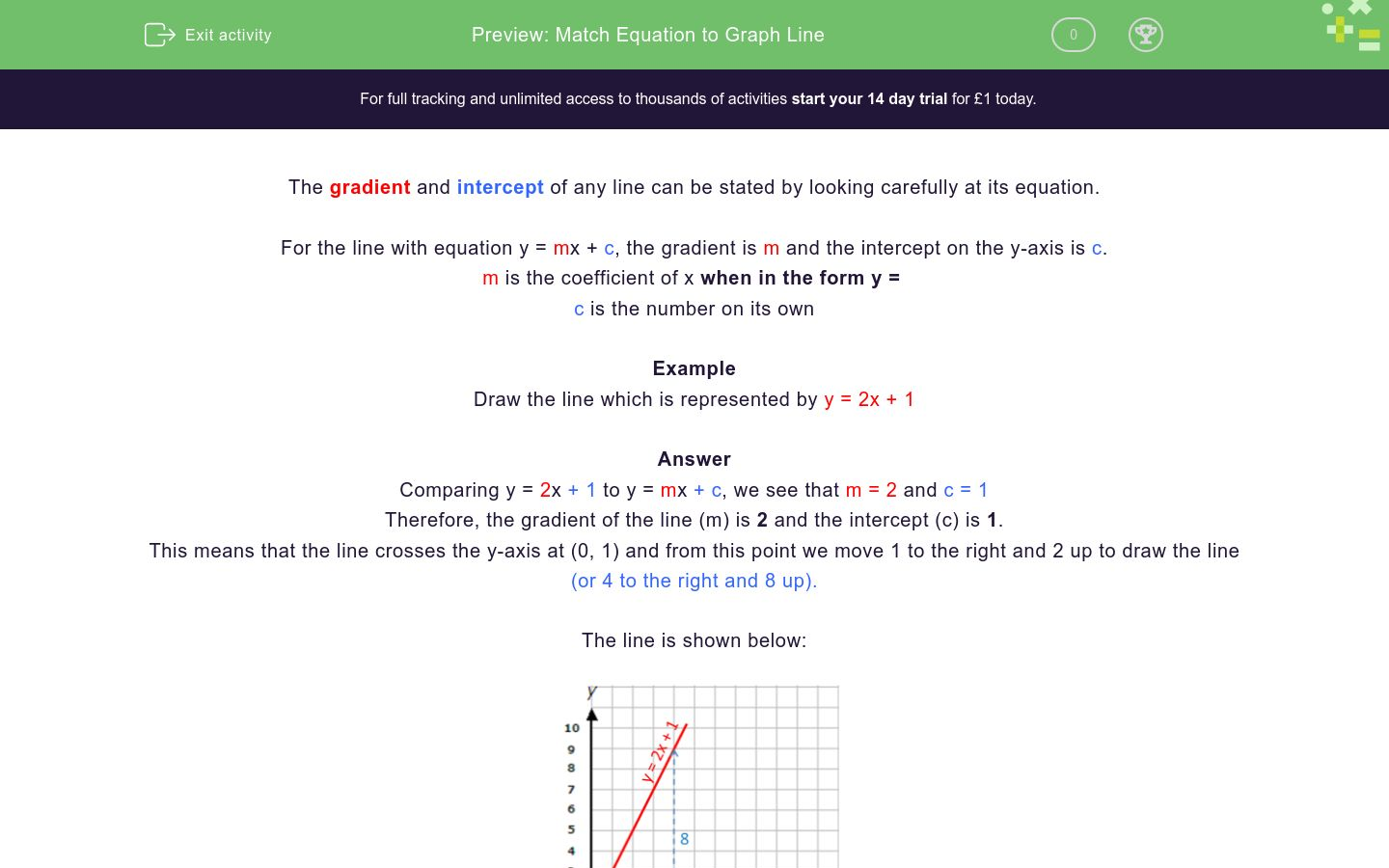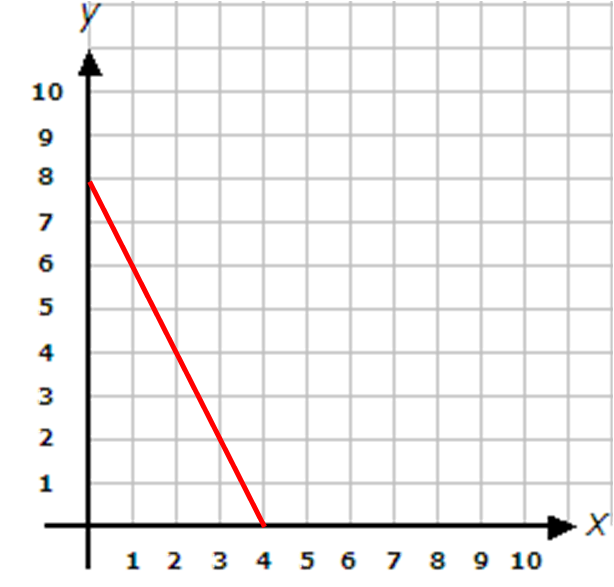# Which Graph Line? (1)

In this worksheet, students match the equation to the correct graph line.Key stage:  KS 4

Curriculum topic:  Algebra

Curriculum subtopic:  Find the Equation of a Line

Difficulty level:### QUESTION 1 of 10

The gradient and intercept of a line can easily be stated by looking carefully at its equation.

For the line with equation y = mx + c, the gradient is m and the intercept on

the y-axis is c.

m is the coefficient of x when in the form y = ......

c is the number on its own

Example

Draw the line which is represented by y = 2x + 1

Answer

Comparing y = 2x + 1 to y = mx + c, we see that m = 2 and c = 1

The gradient of the line, m, is 2 and the intercept, c, is 1.

This means that it crosses the y-axis at (0, 1) and from this point we move 1 to the right and 2 up to draw the line (or 4 to the right and 8 up)

The line is shown below.Which of the given equations represents the red line shown below?y = 2x - 3

x = 2y + 3

y = 2x + 3

y = 3x + 2

Which of the given equations represents the red line shown below?y = 4x - 3

x = ¾y + 4

y = ½x + 4

y = ¾x + 4

Which of the given equations represents the red line shown below?y = 8 - 2x

x = 8x - 2

y = -8x -2

y = 8x + 2

Which of the given equations represents the red line shown below?x = 7y

x = 7

y = 7x

y = 7

Which of the given equations represents the red line shown below?x = 8

x = 8y

y = 8x

y = 8

Which of the given equations represents the red line shown below?x = 0

xy

y = x

y = xy

Which of the given equations represents the red line shown below?x = 8

y = 8 - x

y = 8

y = 8xy

Which of the given equations represents the red line shown below?y = 2x + 6

x = ½y + 4

y = ½x + 6

y = 6x + ½

Which of the given equations represents the red line shown below?y = 2x - 6

x = 2y + 6

y = -2x + 6

y = 6 - ½x

Which of the given equations represents the red line shown below?y = ¼x - 9

x = 9y + ¼

y = -¼x + 9

y = 9 + ¼x

• Question 1

Which of the given equations represents the red line shown below?CORRECT ANSWER
y = 2x + 3
EDDIE SAYS
m = 2 and c = 3
• Question 2

Which of the given equations represents the red line shown below?CORRECT ANSWER
y = ¾x + 4
EDDIE SAYS
m = ¾ and c = 4
• Question 3

Which of the given equations represents the red line shown below?CORRECT ANSWER
y = 8 - 2x
EDDIE SAYS
m = -2 and c = 8
• Question 4

Which of the given equations represents the red line shown below?CORRECT ANSWER
y = 7
EDDIE SAYS
m = 0 and c = 7
• Question 5

Which of the given equations represents the red line shown below?CORRECT ANSWER
x = 8
• Question 6

Which of the given equations represents the red line shown below?CORRECT ANSWER
y = x
EDDIE SAYS
m = 1 and c = 0
• Question 7

Which of the given equations represents the red line shown below?CORRECT ANSWER
y = 8 - x
EDDIE SAYS
m = -1 and c = 8
• Question 8

Which of the given equations represents the red line shown below?CORRECT ANSWER
y = ½x + 6
EDDIE SAYS
m = ½ and c = 6
• Question 9

Which of the given equations represents the red line shown below?CORRECT ANSWER
y = 6 - ½x
EDDIE SAYS
m = -½ and c = 6
• Question 10

Which of the given equations represents the red line shown below?CORRECT ANSWER
y = -¼x + 9
EDDIE SAYS
m = -¼ and c = 9
---- OR ----

Sign up for a £1 trial so you can track and measure your child's progress on this activity.

### What is EdPlace?

We're your National Curriculum aligned online education content provider helping each child succeed in English, maths and science from year 1 to GCSE. With an EdPlace account you’ll be able to track and measure progress, helping each child achieve their best. We build confidence and attainment by personalising each child’s learning at a level that suits them.

Start your £1 trial